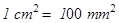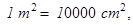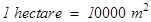### Perimeter and Area - Revision Notes

CBSE Class–VII Subject Mathematics
Revision Notes

Chapter –11
Perimeter and Area

• Perimeter is the distance around a closed figure whereas area is the part of plane occupied by the closed figure.
• Area is the measure of the part of plane or region enclosed by it.
• We have learnt how to find perimeter and area of a square and rectangle in the earlier class. They are:
(a) Perimeter of a square = 4 × side
(b) Perimeter of a rectangle = 2 × (length + breadth)
(c) Area of a square = side × side
(d) Area of a rectangle = length × breadth
• Area of a parallelogram = base × height
• Area of a triangle = $\frac{1}{2}$(area of the parallelogram generated from it)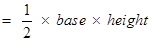• Area of equilateral triangle =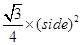• The distance around a circular region is known as its circumference.
• The ratio of circumference and diameter of a circle is a constant is denoted by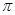(pi).
• Circumference of a circle = πd, where d is the diameter of a circle and  (approximately).
• Area of a circle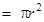,  where r is the radius of the circle.
• Based on the conversion of units for lengths, studied earlier, the units of areas can also be converted: